# Ratio and proportion RATIO compares part to part

• Slides: 24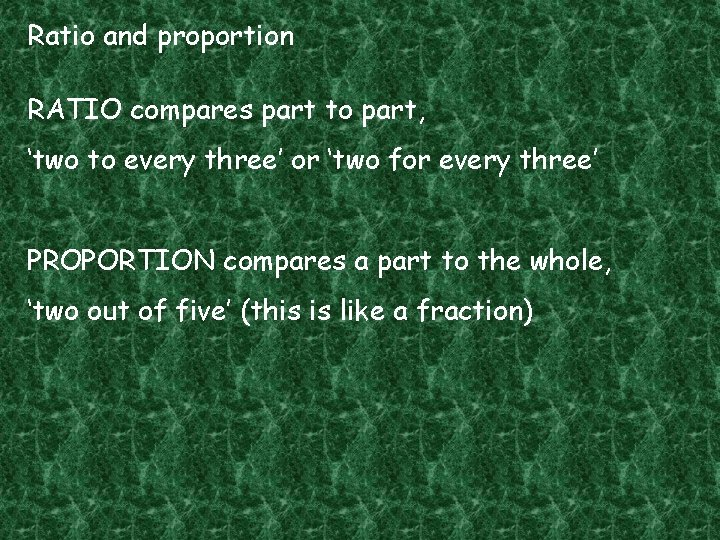Ratio and proportion RATIO compares part to part, ‘two to every three’ or ‘two for every three’ PROPORTION compares a part to the whole, ‘two out of five’ (this is like a fraction)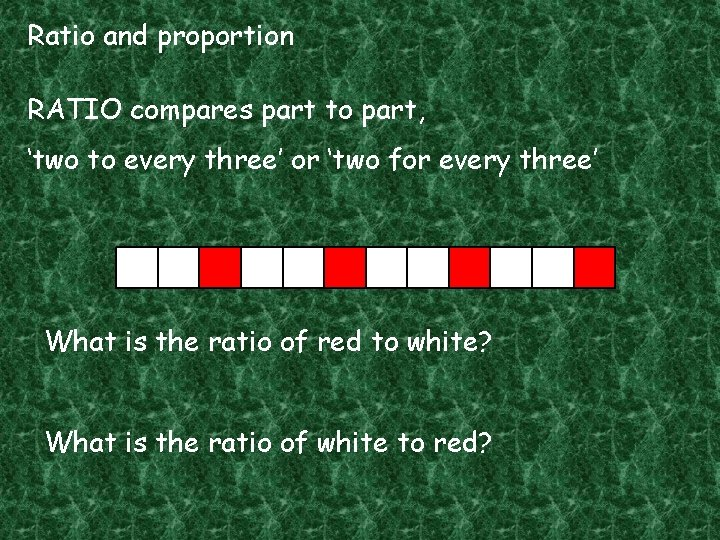Ratio and proportion RATIO compares part to part, ‘two to every three’ or ‘two for every three’ What is the ratio of red to white? What is the ratio of white to red?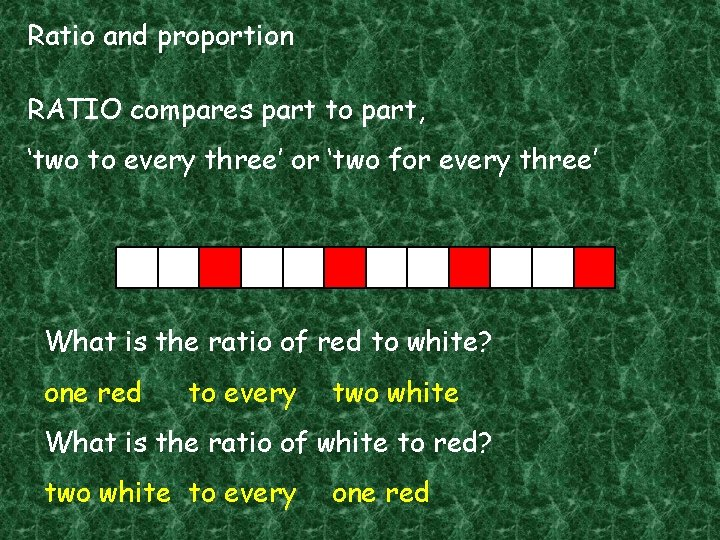Ratio and proportion RATIO compares part to part, ‘two to every three’ or ‘two for every three’ What is the ratio of red to white? one red to every two white What is the ratio of white to red? two white to every one redRatio and proportion RATIO compares part to part, ‘two to every three’ or ‘two for every three’ What is the ratio of red to white? one to two What is the ratio of white to red? two to one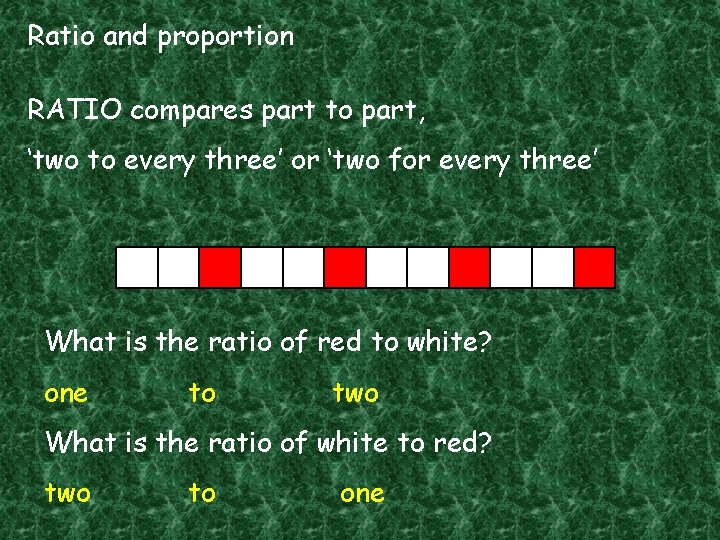Ratio and proportion RATIO compares part to part, ‘two to every three’ or ‘two for every three’ What is the ratio of red to white? one to two What is the ratio of white to red? two to one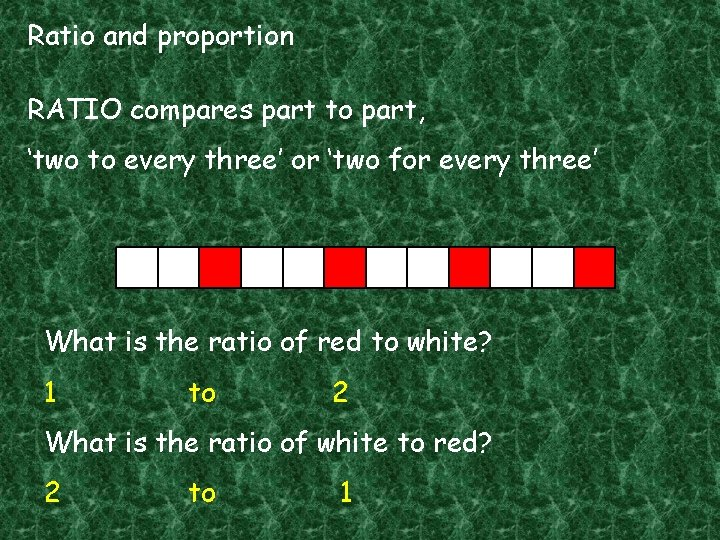Ratio and proportion RATIO compares part to part, ‘two to every three’ or ‘two for every three’ What is the ratio of red to white? 1 to 2 What is the ratio of white to red? 2 to 1Ratio and proportion RATIO compares part to part, ‘two to every three’ or ‘two for every three’ What is the ratio of red to white? 1 : 2 What is the ratio of white to red? 2 : 1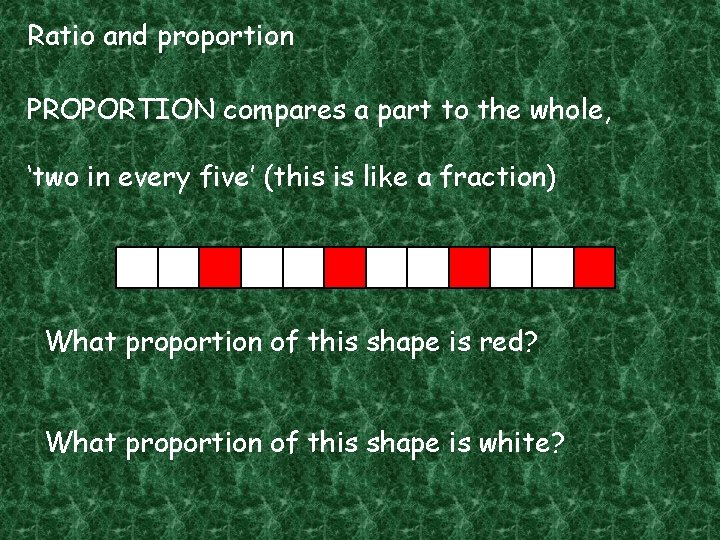Ratio and proportion PROPORTION compares a part to the whole, ‘two in every five’ (this is like a fraction) What proportion of this shape is red? What proportion of this shape is white?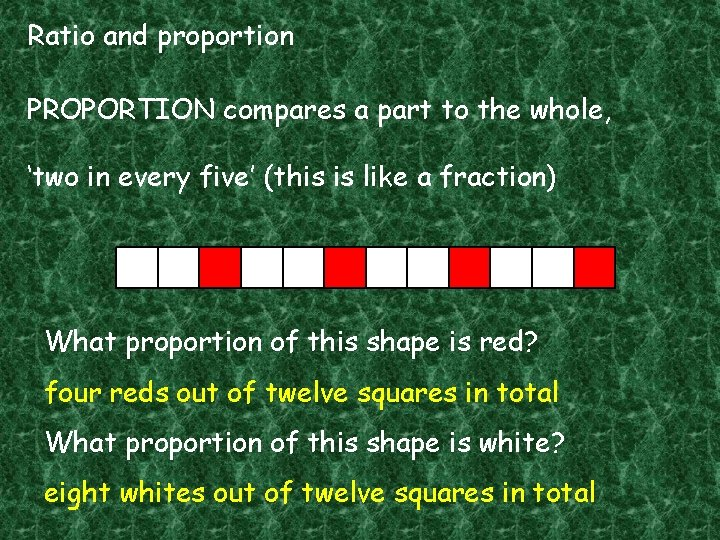Ratio and proportion PROPORTION compares a part to the whole, ‘two in every five’ (this is like a fraction) What proportion of this shape is red? four reds out of twelve squares in total What proportion of this shape is white? eight whites out of twelve squares in totalRatio and proportion PROPORTION compares a part to the whole, ‘two in every five’ (this is like a fraction) What proportion of this shape is red? four out of twelve What proportion of this shape is white? eight out of twelve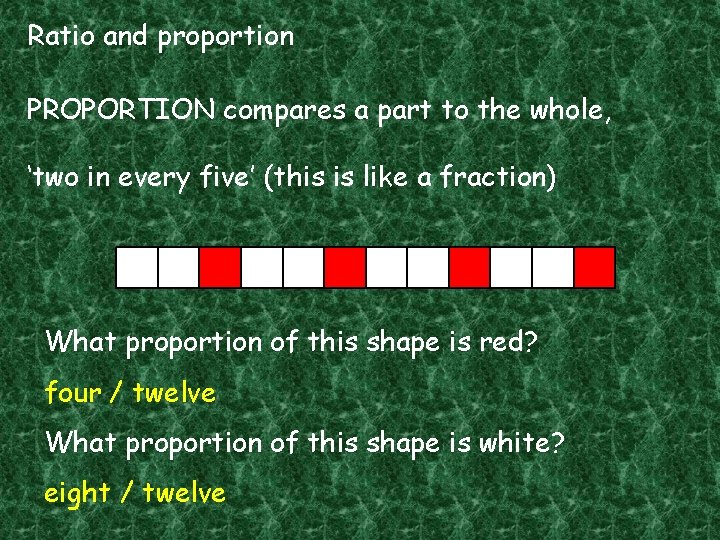Ratio and proportion PROPORTION compares a part to the whole, ‘two in every five’ (this is like a fraction) What proportion of this shape is red? four / twelve What proportion of this shape is white? eight / twelve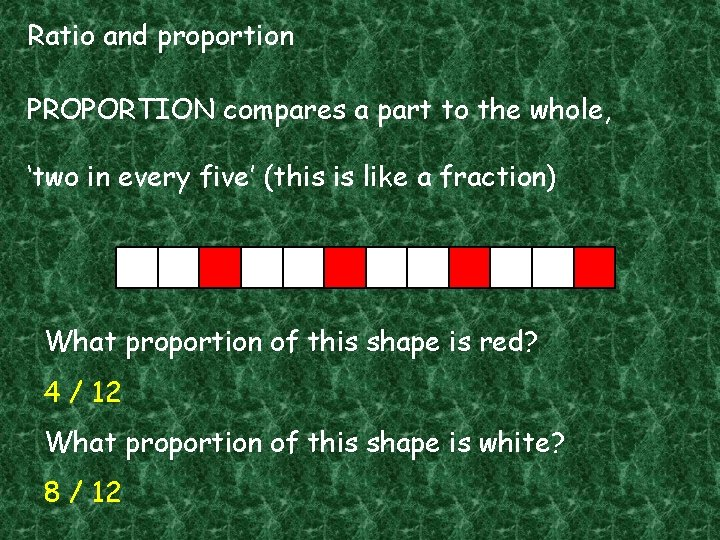Ratio and proportion PROPORTION compares a part to the whole, ‘two in every five’ (this is like a fraction) What proportion of this shape is red? 4 / 12 What proportion of this shape is white? 8 / 12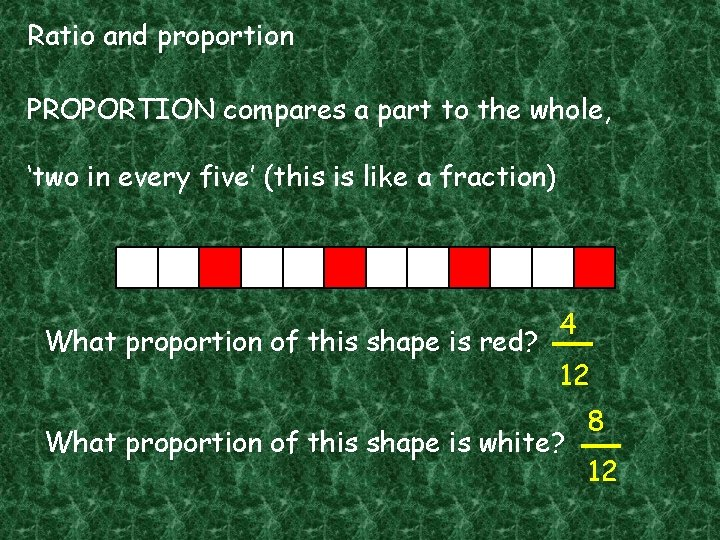Ratio and proportion PROPORTION compares a part to the whole, ‘two in every five’ (this is like a fraction) What proportion of this shape is red? 4 12 What proportion of this shape is white? 8 12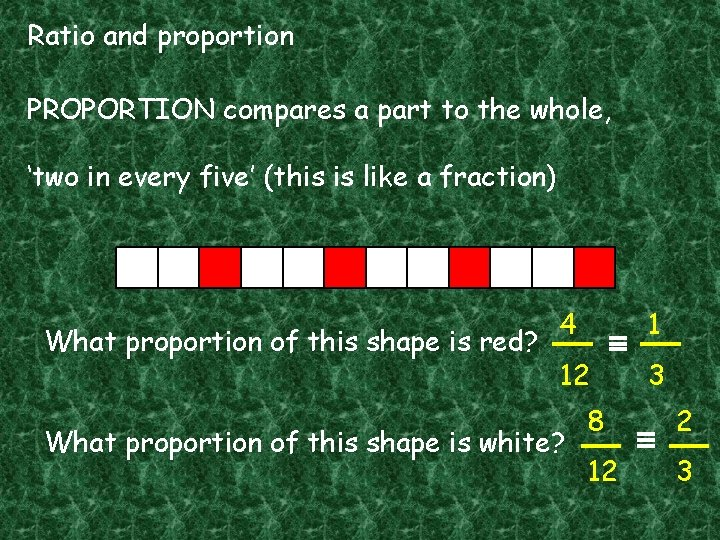Ratio and proportion PROPORTION compares a part to the whole, ‘two in every five’ (this is like a fraction) What proportion of this shape is red? 4 1 12 3 What proportion of this shape is white? 8 2 12 3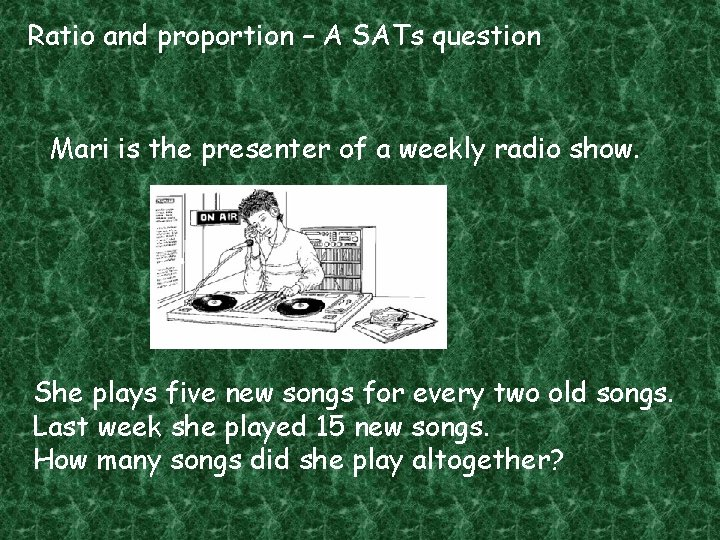Ratio and proportion – A SATs question Mari is the presenter of a weekly radio show. She plays five new songs for every two old songs. Last week she played 15 new songs. How many songs did she play altogether?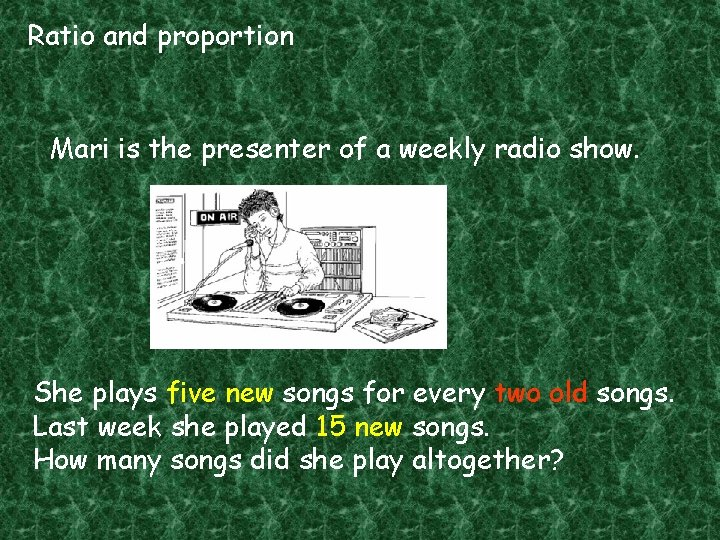Ratio and proportion Mari is the presenter of a weekly radio show. She plays five new songs for every two old songs. Last week she played 15 new songs. How many songs did she play altogether?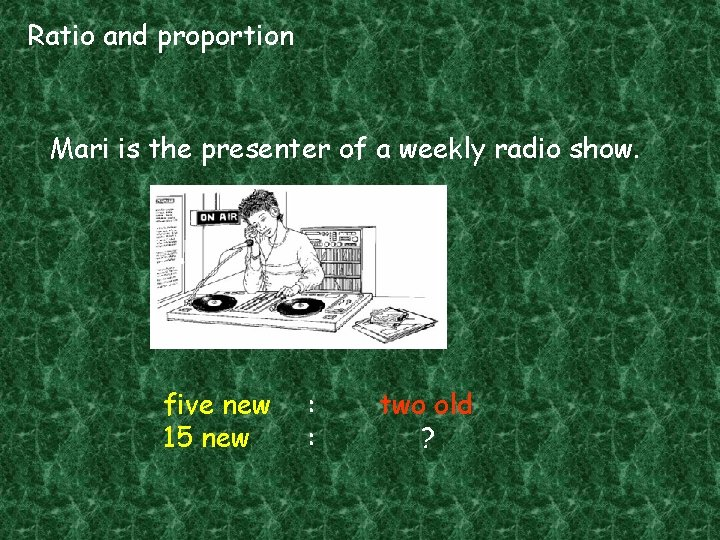Ratio and proportion Mari is the presenter of a weekly radio show. five new 15 new : : two old ?Ratio and proportion Mari is the presenter of a weekly radio show. 5 new 15 new : : 2 old ?Ratio and proportion Mari is the presenter of a weekly radio show. 5: 2 15 : ?Ratio and proportion Mari is the presenter of a weekly radio show. 5: 2 X 3 15 : ?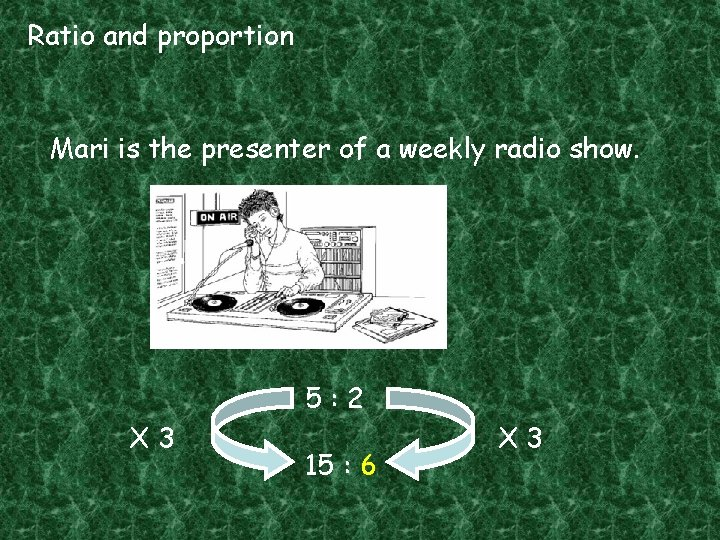Ratio and proportion Mari is the presenter of a weekly radio show. 5: 2 X 3 15 : 6 X 3Ratio and proportion Mari is the presenter of a weekly radio show. How many songs did she play altogether? She played 6 old songs and 15 new songs.Ratio and proportion Mari is the presenter of a weekly radio show. How many songs did she play altogether? She played 21 songs altogether.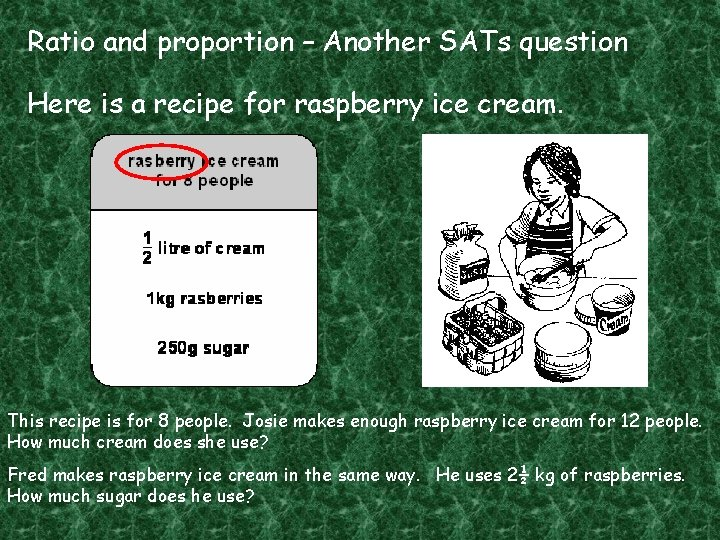Ratio and proportion – Another SATs question Here is a recipe for raspberry ice cream. This recipe is for 8 people. Josie makes enough raspberry ice cream for 12 people. How much cream does she use? Fred makes raspberry ice cream in the same way. He uses 2½ kg of raspberries. How much sugar does he use?